OpenGV A library for solving calibrated central and non-central geometric vision problemsopengv::relative_pose Namespace Reference

The namespace for the relative pose methods. More...

## Classes

class  MACentralRelative

class  MANoncentralRelative

class  MANoncentralRelativeMulti

## Functions

translation_t twopt (const RelativeAdapterBase &adapter, bool unrotate, const std::vector< int > &indices)
Compute the translation between two central viewpoints with known relative rotation, and using two correspondences. More...

Compute the translation between two central viewpoints with known relative rotation, and using two correspondences. More...

Compute the rotation between two central viewpoints with pure rotation change, using only two correspondences. More...

Compute the rotation between two central viewpoints with pure rotation change, using only two correspondences. More...

Compute the rotation between two central viewpoints with pure rotation change using Arun's method . Using all available correspondences. More...

Compute the rotation between two central viewpoints with pure rotation change using Arun's method . More...

Compute the complex essential matrices between two central viewpoints using Stewenius' method . Using all available correspondences. More...

Compute the complex essential matrices between two central viewpoints using Stewenius' method . More...

Compute the essential matrices between two central viewpoints using Nister's method . Using all available correspondences. More...

Compute the essential matrices between two central viewpoints using Nister's method . More...

Compute the rotation matrices between two central viewpoints using Kneips's method . Only minimal case. More...

Compute the essential matrices between two central viewpoints using the seven-point algorithm . Using all available correspondences. More...

Compute the essential matrices between two central viewpoints using the seven-point algorithm . More...

Compute the essential matrix between two central viewpoints using the eight-point algorithm [9,10]. Using all available correspondences. More...

Compute the essential matrix between two central viewpoints using the eight-point algorithm [9,10]. More...

Compute the rotation matrix between two central viewpoints as an iterative eigenproblem . Using all available correspondences. More...

rotation_t eigensolver (const RelativeAdapterBase &adapter, const std::vector< int > &indices, eigensolverOutput_t &output, bool useWeights=false)
Compute the rotation matrix between two central viewpoints as an iterative eigenproblem . More...

Compute the rotation matrix between two central viewpoints as an iterative eigenproblem . Using all available correspondences. Outputs only the rotation. More...

rotation_t eigensolver (const RelativeAdapterBase &adapter, const std::vector< int > &indices, bool useWeights=false)
Compute the rotation matrix between two central viewpoints as an iterative eigenproblem . Outputs only the rotation. More...

Compute the relative rotation between two non-central viewpoints following Stewenius' method . Assuming exactly 6 correspondences. More...

Compute the relative rotation between two non-central viewpoints following Stewenius' method using 6 correspondences . More...

Compute the rotation matrix between two non-central viewpoints as an iterative eigenproblem. Using all available correspondences. More...

rotation_t ge (const RelativeAdapterBase &adapter, const std::vector< int > &indices, geOutput_t &output, bool useWeights=false)
Compute the rotation matrix between two non-central viewpoints as an iterative eigenproblem. More...

Compute the rotation matrix between two non-central viewpoints as an iterative eigenproblem. Using all available correspondences. Outputs only the rotation. More...

rotation_t ge (const RelativeAdapterBase &adapter, const std::vector< int > &indices, bool useWeights=false)
Compute the rotation matrix between two non-central viewpoints as an iterative eigenproblem. Outputs only the rotation. More...

Compute the relative pose between two non-central viewpoints following Li's method . Using all available correspondences. More...

Compute the relative pose between two non-central viewpoints following Li's method . More...

Compute the pose between two viewpoints using nonlinear optimization. Using all available correspondences. Works for both central and non-central case. More...

Compute the pose between two viewpoints using nonlinear optimization. Works for both central and non-central case. More...

## Detailed Description

The namespace for the relative pose methods.

## Function Documentation

 rotation_t opengv::relative_pose::eigensolver ( const RelativeAdapterBase & adapter, eigensolverOutput_t & output, bool useWeights = false )

Compute the rotation matrix between two central viewpoints as an iterative eigenproblem . Using all available correspondences.

Parameters
 [in] adapter Visitor holding bearing-vector correspondences, plus the initial rotation for the optimization. [out] output Returns more complete information (position of viewpoint 2 seen from viewpoint 1, eigenvectors and eigenvalues) [in] useWeights Use weights to weight the summation terms?
Returns
Rotation matrix from viewpoint 2 to viewpoint 1.
 rotation_t opengv::relative_pose::eigensolver ( const RelativeAdapterBase & adapter, const std::vector< int > & indices, eigensolverOutput_t & output, bool useWeights = false )

Compute the rotation matrix between two central viewpoints as an iterative eigenproblem .

Parameters
 [in] adapter Visitor holding bearing-vector correspondences, plus the initial rotation for the optimization. [in] indices Indices of the correspondences used for deriving the rotation matrix. [out] output Returns more complete information (position of viewpoint 2 seen from viewpoint 1, eigenvectors and eigenvalues) [in] useWeights Use weights to weight the summation terms?
Returns
Rotation matrix from viewpoint 2 to viewpoint 1.
 rotation_t opengv::relative_pose::eigensolver ( const RelativeAdapterBase & adapter, bool useWeights = false )

Compute the rotation matrix between two central viewpoints as an iterative eigenproblem . Using all available correspondences. Outputs only the rotation.

Parameters
 [in] adapter Visitor holding bearing-vector correspondences, plus the initial rotation for the optimization. [in] useWeights Use weights to weight the summation terms?
Returns
Rotation matrix from viewpoint 2 to viewpoint 1.
 rotation_t opengv::relative_pose::eigensolver ( const RelativeAdapterBase & adapter, const std::vector< int > & indices, bool useWeights = false )

Compute the rotation matrix between two central viewpoints as an iterative eigenproblem . Outputs only the rotation.

Parameters
 [in] adapter Visitor holding bearing-vector correspondences, plus the initial rotation for the optimization. [in] indices Indices of the correspondences used for deriving the rotation matrix. [in] useWeights Use weights to weight the summation terms?
Returns
Rotation matrix from viewpoint 2 to viewpoint 1.

Compute the essential matrix between two central viewpoints using the eight-point algorithm [9,10]. Using all available correspondences.

Parameters
 [in] adapter Visitor holding bearing-vector correspondences.
Returns
Real essential matrix (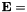skew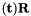, where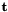is the position of viewpoint 2 seen from viewpoint 1, and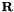is the rotation from viewpoint 2 back to viewpoint 1).
 essential_t opengv::relative_pose::eightpt ( const RelativeAdapterBase & adapter, const std::vector< int > & indices )

Compute the essential matrix between two central viewpoints using the eight-point algorithm [9,10].

Parameters
 [in] adapter Visitor holding bearing-vector correspondences. [in] indices Indices of the correspondences used for deriving the essential matrix.
Returns
Real essential matrix (skew, whereis the position of viewpoint 2 seen from viewpoint 1, andis the rotation from viewpoint 2 back to viewpoint 1).
 rotations_t opengv::relative_pose::fivept_kneip ( const RelativeAdapterBase & adapter, const std::vector< int > & indices )

Compute the rotation matrices between two central viewpoints using Kneips's method . Only minimal case.

Parameters
 [in] adapter Visitor holding bearing-vector correspondences. [in] indices Indices of the correspondences used for deriving the rotation matrices.
Returns
Rotation matrices from viewpoint 2 to viewpoint 1 (maximum 20 solutions).

Compute the essential matrices between two central viewpoints using Nister's method . Using all available correspondences.

Parameters
 [in] adapter Visitor holding bearing-vector correspondences.
Returns
Real essential matrices (skew, whereis the position of viewpoint 2 seen from viewpoint 1, andis the rotation from viewpoint 2 back to viewpoint 1, maximum of 10 solutions).
 essentials_t opengv::relative_pose::fivept_nister ( const RelativeAdapterBase & adapter, const std::vector< int > & indices )

Compute the essential matrices between two central viewpoints using Nister's method .

Parameters
 [in] adapter Visitor holding bearing-vector correspondences. [in] indices Indices of the correspondences used for deriving the essential matrices.
Returns
Real essential matrices (skew, whereis the position of viewpoint 2 seen from viewpoint 1, andis the rotation from viewpoint 2 back to viewpoint 1, maximum of 10 solutions).

Compute the complex essential matrices between two central viewpoints using Stewenius' method . Using all available correspondences.

Parameters
 [in] adapter Visitor holding bearing-vector correspondences.
Returns
Complex essential matrices (skew, whereis the position of viewpoint 2 seen from viewpoint 1, andis the rotation from viewpoint 2 back to viewpoint 1, maximum of 10 solutions).
 complexEssentials_t opengv::relative_pose::fivept_stewenius ( const RelativeAdapterBase & adapter, const std::vector< int > & indices )

Compute the complex essential matrices between two central viewpoints using Stewenius' method .

Parameters
 [in] adapter Visitor holding bearing-vector correspondences. [in] indices Indices of the correspondences used for deriving the essential matrices.
Returns
Complex essential matrices (skew, whereis the position of viewpoint 2 seen from viewpoint 1, andis the rotation from viewpoint 2 back to viewpoint 1, maximum of 10 solutions).
 rotation_t opengv::relative_pose::ge ( const RelativeAdapterBase & adapter, geOutput_t & output, bool useWeights = false )

Compute the rotation matrix between two non-central viewpoints as an iterative eigenproblem. Using all available correspondences.

Parameters
 [in] adapter Visitor holding bearing-vector correspondences, the multi- camera configuration, plus the initial rotation for the optimization. [out] output Returns more complete information (position of viewpoint 2 seen from viewpoint 1, eigenvectors and eigenvalues) [in] useWeights Use weights to weight the summation terms?
Returns
Rotation matrix from viewpoint 2 to viewpoint 1.
 rotation_t opengv::relative_pose::ge ( const RelativeAdapterBase & adapter, const std::vector< int > & indices, geOutput_t & output, bool useWeights = false )

Compute the rotation matrix between two non-central viewpoints as an iterative eigenproblem.

Parameters
 [in] adapter Visitor holding bearing-vector correspondences, the multi- camera configuration, plus the initial rotation for the optimization. [in] indices Indices of the correspondences used for deriving the rotation matrix. [out] output Returns more complete information (position of viewpoint 2 seen from viewpoint 1, eigenvectors and eigenvalues) [in] useWeights Use weights to weight the summation terms?
Returns
Rotation matrix from viewpoint 2 to viewpoint 1.
 rotation_t opengv::relative_pose::ge ( const RelativeAdapterBase & adapter, bool useWeights = false )

Compute the rotation matrix between two non-central viewpoints as an iterative eigenproblem. Using all available correspondences. Outputs only the rotation.

Parameters
 [in] adapter Visitor holding bearing-vector correspondences, the multi- camera configuration, plus the initial rotation for the optimization. [in] useWeights Use weights to weight the summation terms?
Returns
Rotation matrix from viewpoint 2 to viewpoint 1.
 rotation_t opengv::relative_pose::ge ( const RelativeAdapterBase & adapter, const std::vector< int > & indices, bool useWeights = false )

Compute the rotation matrix between two non-central viewpoints as an iterative eigenproblem. Outputs only the rotation.

Parameters
 [in] adapter Visitor holding bearing-vector correspondences, the multi- camera configuration, plus the initial rotation for the optimization. [in] indices Indices of the correspondences used for deriving the rotation matrix. [in] useWeights Use weights to weight the summation terms?
Returns
Rotation matrix from viewpoint 2 to viewpoint 1.

Compute the pose between two viewpoints using nonlinear optimization. Using all available correspondences. Works for both central and non-central case.

Parameters
 [in] adapter Visitor holding bearing-vector correspondences, the multi-camera configuration, plus the initial values.
Returns
Pose of viewpoint 2 seen from viewpoint 1 (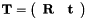, withbeing the position of viewpoint 2 seen from viewpoint 1, andbeing the rotation from viewpoint 2 to viewpoint 1).
 transformation_t opengv::relative_pose::optimize_nonlinear ( RelativeAdapterBase & adapter, const std::vector< int > & indices )

Compute the pose between two viewpoints using nonlinear optimization. Works for both central and non-central case.

Parameters
 [in] adapter Visitor holding bearing-vector correspondences, the multi-camera configuration, plus the initial values. [in] indices Indices of the correspondences used for deriving the pose.
Returns
Pose of viewpoint 2 seen from viewpoint 1 (, withbeing the position of viewpoint 2 seen from viewpoint 1, andbeing the rotation from viewpoint 2 to viewpoint 1).

Compute the rotation between two central viewpoints with pure rotation change using Arun's method . Using all available correspondences.

Parameters
 [in] adapter Visitor holding bearing-vector correspondences.
Returns
Rotation from viewpoint 2 to viewpoint 1.
 rotation_t opengv::relative_pose::rotationOnly ( const RelativeAdapterBase & adapter, const std::vector< int > & indices )

Compute the rotation between two central viewpoints with pure rotation change using Arun's method .

Parameters
 [in] adapter Visitor holding bearing-vector correspondences. [in] indices Indices of the correspondences used for deriving the rotation.
Returns
Rotation from viewpoint 2 to viewpoint 1.

Compute the essential matrices between two central viewpoints using the seven-point algorithm . Using all available correspondences.

Parameters
 [in] adapter Visitor holding bearing-vector correspondences.
Returns
Real essential matrices (skew, whereis the position of viewpoint 2 seen from viewpoint 1, andis the rotation from viewpoint 2 back to viewpoint 1, maximum 3 solutions).
 essentials_t opengv::relative_pose::sevenpt ( const RelativeAdapterBase & adapter, const std::vector< int > & indices )

Compute the essential matrices between two central viewpoints using the seven-point algorithm .

Parameters
 [in] adapter Visitor holding bearing-vector correspondences. [in] indices Indices of the correspondences used for deriving the essential matrices.
Returns
Real essential matrices (skew, whereis the position of viewpoint 2 seen from viewpoint 1, andis the rotation from viewpoint 2 back to viewpoint 1, maximum 3 solutions).

Compute the relative pose between two non-central viewpoints following Li's method . Using all available correspondences.

Parameters
 [in] adapter Visitor holding bearing-vector correspondences, plus the multi-camera configuration.
Returns
Pose of viewpoint 2 seen from viewpoint 1 (, withbeing the position of viewpoint 2 seen from viewpoint 1, andbeing the rotation from viewpoint 2 to viewpoint 1).
 transformation_t opengv::relative_pose::seventeenpt ( const RelativeAdapterBase & adapter, const std::vector< int > & indices )

Compute the relative pose between two non-central viewpoints following Li's method .

Parameters
 [in] adapter Visitor holding bearing-vector correspondences, plus the multi-camera configuration. [in] indices Indices of the correspondences used for deriving the rotation matrix.
Returns
Pose of viewpoint 2 seen from viewpoint 1 (, withbeing the position of viewpoint 2 seen from viewpoint 1, andbeing the rotation from viewpoint 2 to viewpoint 1).

Compute the relative rotation between two non-central viewpoints following Stewenius' method . Assuming exactly 6 correspondences.

Parameters
 [in] adapter Visitor holding bearing-vector correspondences, plus the multi-camera configuration.
Returns
Rotations from viewpoint 2 back to viewpoint 1
 rotations_t opengv::relative_pose::sixpt ( const RelativeAdapterBase & adapter, const std::vector< int > & indices )

Compute the relative rotation between two non-central viewpoints following Stewenius' method using 6 correspondences .

Parameters
 [in] adapter Visitor holding bearing-vector correspondences, plus the multi-camera configuration. [in] indices Indices of the six correspondences used for deriving the rotation matrix.
Returns
Rotations from viewpoint 2 back to viewpoint 1
 translation_t opengv::relative_pose::twopt ( const RelativeAdapterBase & adapter, bool unrotate, const std::vector< int > & indices )

Compute the translation between two central viewpoints with known relative rotation, and using two correspondences.

Parameters
 [in] adapter Visitor holding bearing-vector correspondences and known relative rotation. [in] unrotate Set to true if known rotation should be used to unrotate bearing vectors from viewpoint 2 (not required in case of identity rotation). [in] indices Indices of the two correspondences used for deriving the translation.
Returns
Position of viewpoint 2 seen from viewpoint 1.
 translation_t opengv::relative_pose::twopt ( const RelativeAdapterBase & adapter, bool unrotate, size_t index0 = 0, size_t index1 = 1 )

Compute the translation between two central viewpoints with known relative rotation, and using two correspondences.

Parameters
 [in] adapter Visitor holding bearing-vector correspondences and known relative rotation. [in] unrotate Set to true if known rotation should be used to unrotate bearing vectors from viewpoint 2 (not required in case of identity rotation). [in] index0 Index of the first correspondence used for deriving the translation (use default value if only two provided anyway). [in] index1 Index of the second correspondence used for deriving the translation (use default vector if only two provided anyway).
Returns
Position of viewpoint 2 seen from viewpoint 1.
 rotation_t opengv::relative_pose::twopt_rotationOnly ( const RelativeAdapterBase & adapter, const std::vector< int > & indices )

Compute the rotation between two central viewpoints with pure rotation change, using only two correspondences.

Parameters
 [in] adapter Visitor holding bearing-vector correspondences. [in] indices Indices of the two correspondences used for deriving the rotation.
Returns
Rotation from viewpoint 2 to viewpoint 1.
 rotation_t opengv::relative_pose::twopt_rotationOnly ( const RelativeAdapterBase & adapter, size_t index0 = 0, size_t index1 = 1 )

Compute the rotation between two central viewpoints with pure rotation change, using only two correspondences.

Parameters
 [in] adapter Visitor holding bearing-vector correspondences. [in] index0 Index of the first correspondence used for deriving the rotation (use default value if only two provided anyway). [in] index1 Index of the second correspondence used for deriving the rotation (use default value if only two provided anyway).
Returns
Rotation from viewpoint 2 to viewpoint 1.